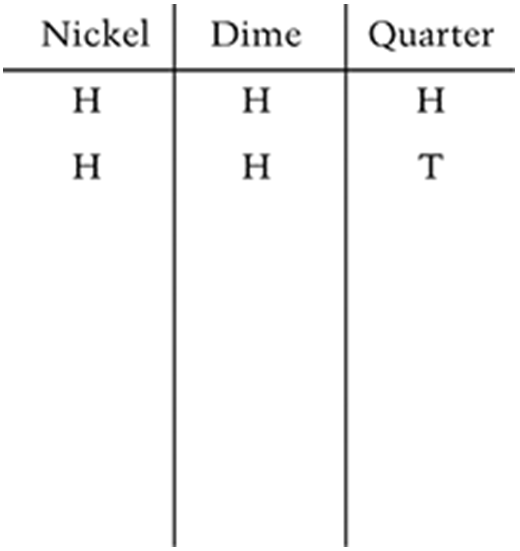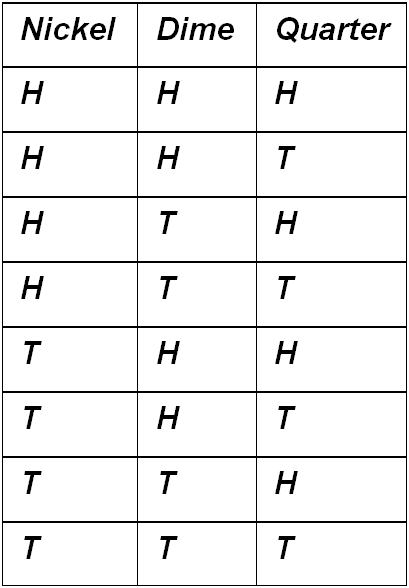# Statistics and Probability / Probability Diagrams and Tables

Activity 13 of 24
In this lesson, students develop a process for determining the probability of two independent events occurring at the same time.

## Planning and Resources

Objectives
Students distinguish between an experiment in which the outcomes are equally likely and one in which the outcomes are not equally likely. They organize a list of all possible outcomes and find the probability of a certain outcome for a compound event.

Vocabulary
probability
compound events
equally likely
tree diagram

Standard:

## Lesson Snapshot

#### Understanding

The probability of a compound event can be found by listing the outcomes for one event followed by the other, creating a probability tree, or by using the product rule for independent events.

### What to look for

Students should understand when it is appropriate to multiply the probabilities of individual outcomes in order to determine the probability of a compound event.

### Sample Assessment

A nickel, a dime, and a quarter are flipped at the same time. Each coin can land either heads up (H) or tails up (T). List all the different possible outcomes for this event in the chart below. The list has been started for you.